# Functions and Formulas in Excel

### What is Formula & Function in Excel?

A formula is a mathematical relationship or rule expressed in symbols that calculates the value of a cell.

A function is a pre-defined formula available in Excel that is ready to use.

A formula in excel starts with '=' inside a cell.

For example, to show the addition of B2 and B3 value in B4, we have written '=B3+B2' in B4 and hit Enter key. This gives the calculated value to B4.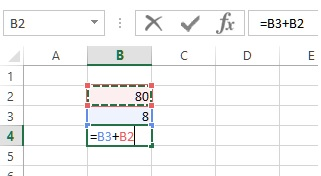The result will be shown like this.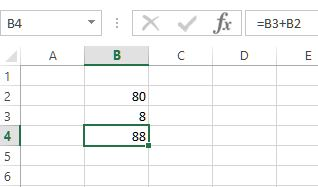If we try to add two string value instead of two integer values, we will get error like shown in the image below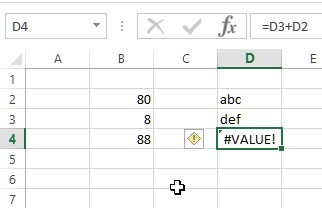#### Operators in Formula

Excel understand the operators in formula as it is treated in normal mathematical calculations. Look at the image below.

In B5, we wrote '=B2+B3*2' and this resulted as 96 because first it multiplied 8 by 2 and then added to 80.

In B5, we wrote '=(C2+C3)*2' and this resulted as 176 because first it added C2 and C3 and then multiplied by 2.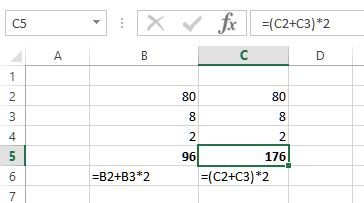Similarly, the precedene of division, additions and substraction work.

### How to display a mathematical or expressive data as string in Excel?

Notice the data in B6 and C6 in above picture; normally when we copy-paste these strings in any cells, Excel tries to evaulate the values of those cells. But what if you just want to display them as it is? In that case, we need to prefix the formula or mathematical expression with single quote (') like displayed in the picture below.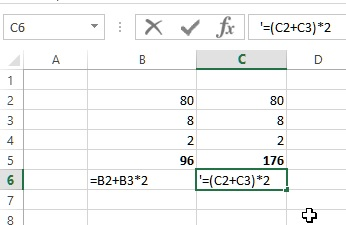The result will be like### How to Copy-Paste a formula to other relevant cells in Excel?

Let's assume a scenario as shown below where we want to copy the formula of D2 to D3, D4 so that we get total of all the records.

There are two ways to copy the formula

1. Go to D2 and Copy (either right click and Copy or press CTRL+C). Now select both D3 and D4 cells and Paste (right click and Paste or CTRL+V).

2. Go to D2 and hold the bottom-right corner of the cell (the mouse cursor becomes + as shown below) and drag to D3 and D4.The result would be like this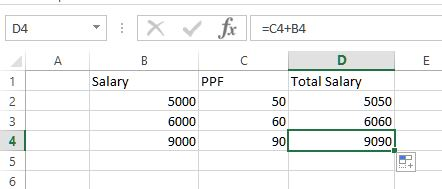### How to quickly know the sum & average of selected cells value in Excel?

It is very easy, just select all the cells you want to know the sum & average of and look at the right side of MS Excel status bar and you would be seeing them like picture below.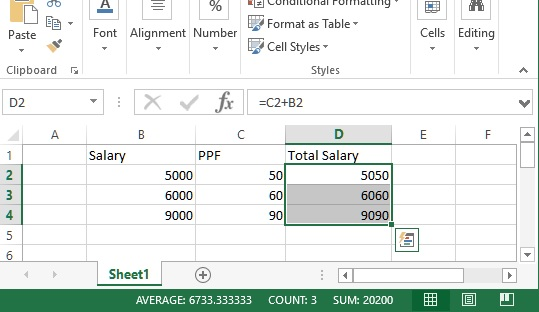Here

1. AVERAGE is the average value of selected cells (D2, D3 & D4)
2. COUNT is the number of cells selected
3. SUM is the sum of selected cells value.

Quickies• Can we add multiple cell value by separating with + symbol (eg. =A1+A2+A3+A4) ? - YES
• Can we add, subtract, multiply and divide at once using cells reference (eg. =A1-A2*A3/A4) ? - YES
• Can we directly add, subtract, multiply and divide numbers in cells (eg. =20+30-20*40/3)? - YES

### How to select range of cells in MS Excel?

Range of cells is the selection of all the cells between starting and ending of the cell. To select range of cells, we separate two cells using colon (:).

For example:

If we want to select B2 to B4, we can write B2:B4. This will select B2, B3 & B4 cells. Generally, range is used within the inbuilt functions of MS Excel.

### How to insert a function in Excel?

To insert an inbuilt function in MS Excel, select a cell and then press Fx icon as shown in the picture below (In this case, function will be inserted into D5 cell).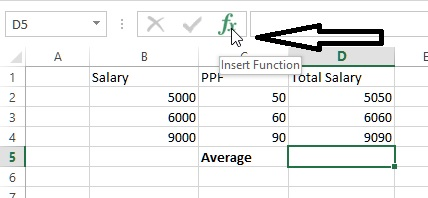A dialog box opens with the list of functions available in MS Excel and by default 'Most Recently Used' function apears in the list. We can change the categroy dropdown to choose a function available in that category.

For this demo, let's use AVERAGE function from the list (In case this function is not available in the default list of your screen, simply use the Search for a function box to search it).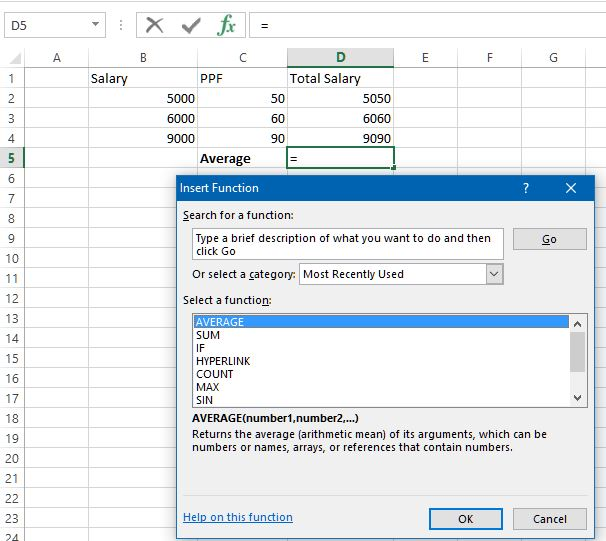After selecting AVERAGE from the list of functions, click OK. This opens another dialog box that ask us the argument to pass into this function.

Average baseically returns the average of its argument. So either we can write all cells one by one in Number1, Number2 etc. boxes or we can specify them by selecting the first cell (D2) and then start dragging (the screen changes as below). Keep dragging till you reach to your target cell (D4). Excel automatically surrounds selected cells with dashed border to notify that those cells have been selected.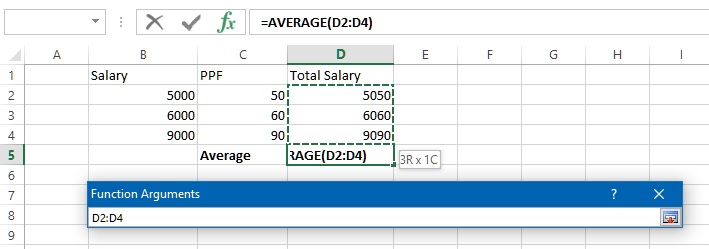We will get the range of cells written in Number1 text box as displayed in below picture. The result also appears on the dialog box. Now click OK.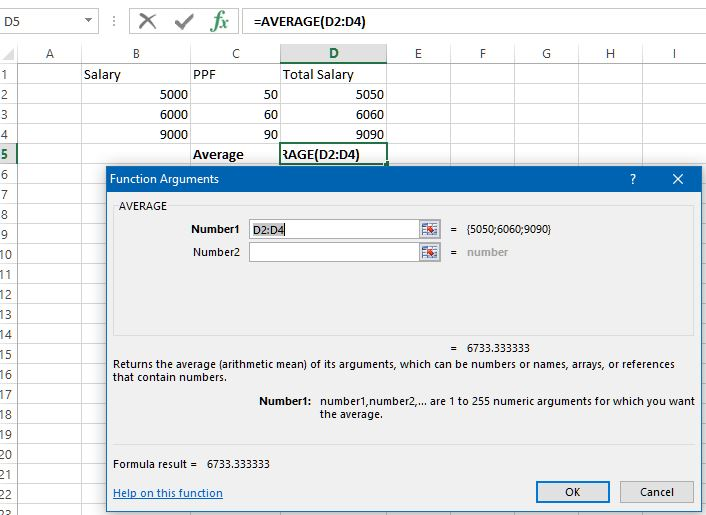and here is the result.Quickies1. Can we write the function directly into the D5 cell? - YES
2. If we can directly write functions into the Cell, why excel has given long steps like above? - All users are not as intelligent as you are...... OK, Actually many functions have more than one arguments and it might be difficult for many to remember those arguments.

Views: 5865 | Post Order: 3